# Getting started with SR-Research EyeLink eye-tracker data

At the Donders we have an Eyelink 1000 eyetracker that supports sampling rates up to 2000Hz for monocular and 1000Hz for binocular tracking. For a technical overview about Eyelink 1000 characteristics look here

The eye-tracker can be used on itself, or in combination with other data acquisition techniques (e.g. EEG, MEG, TMS or fMRI). FieldTrip allows you to analyze the eye-tracker data in all of these situations. In the following I will provide the background of the data acquisition and present some examples on how to analyze eye-tracker data.

With your presentation script on the stimulus presentation computer you can also write “data” to disk in the form of behavioral log files. In the subsequent examples we will not consider integrating the physiological measurements with the “data” in these behavioral log files. We will only consider physiological data from the eye-tracker, from the EEG system and from the MEG system.

## Eye tracker recordings by themselves

It is possible to use the eye-tracker in combination with a behavioral task that involves stimuli (usually on a screen) and response, but without EEG or MEG recordings. In this case the EyeLink computer records all data.

### Converting the EDF file to an ASC file

The Eyelink 1000 output is an _.EDF file (eyelink data file). There are several ways to read (directly or indirectly) this data into MATLAB. To use FieldTrip for data analysis, you have to convert the EDF file to an ASCII text format using the Eyelink executable EDF2ASC.EXE. You need to run the EDF2ASC.EXE that can be downloaded here under MSDOS on the Eyelink computer and find your _.EDF recording as follow

``````EDF2ASC filename.edf
``````

You can type DIR to see if your *.EDF file is located in the current working directory. When the conversion is finished, you need to reboot the Eyelink PC to windows to read your data, because in MSDOS you cannot use USB memory stick.

After converting the EDF file to ASC format and transferring it from the Eyelink computer, we can read it in MATLAB.

To read in the continuous data, you would simply do this:

``````filename_eye = 'tobsta35.asc';

cfg = [];
cfg.dataset = filename_eye;
data_eye = ft_preprocessing(cfg);
``````

The output data contains this

``````disp(data_eye)

hdr: [1x1 struct]
label: {4x1 cell}
time: {[1x3639179 double]}
trial: {[4x3639179 double]}
fsample: 1000
sampleinfo: [1 3639179]
cfg: [1x1 struct]

disp(data_eye.label)

'1'
'2'
'3'
'4'
``````

Channel 1 represents time, channel 2 is the horizontal x-coordinate, channel 3 is the vertical y-coordinate and channel 4 is the pupil dilation.

If you want your channel names to be more consistent, you can use the following montage (see ft_apply_montage) to rename the channels while preprocessing:

``````cfg = [];
cfg.dataset          = filename_eye;
cfg.montage.tra      = eye(4);
cfg.montage.labelorg = {'1', '2', '3', '4'};
cfg.montage.labelnew = {'EYE_TIMESTAMP', 'EYE_HORIZONTAL', 'EYE_VERTICAL', 'EYE_DIAMETER'};
data_eye = ft_preprocessing(cfg);
``````

Typically you would want to analyze the eye movements relative to certain events (e.g. stimuli). For that you would use a trial-based representation, where each trial is time-locked to the event of interest.

The events represented in the eye-tracker datafile can be explored using ft_read_event like this:

``````event_eye = ft_read_event(filename_eye);

disp(unique({event_eye.type}))

'INPUT'
``````

You can see that all events are coded as type “INPUT”. The following plots the event values against the time. Although the dots are very small, there is an individual point for each INPUT event.

``````figure
plot([event_eye.sample]./data_eye.hdr.Fs, [event_eye.value], '.')
title('EYE INPUT')
xlabel('time (s)');
ylabel('trigger value');
``````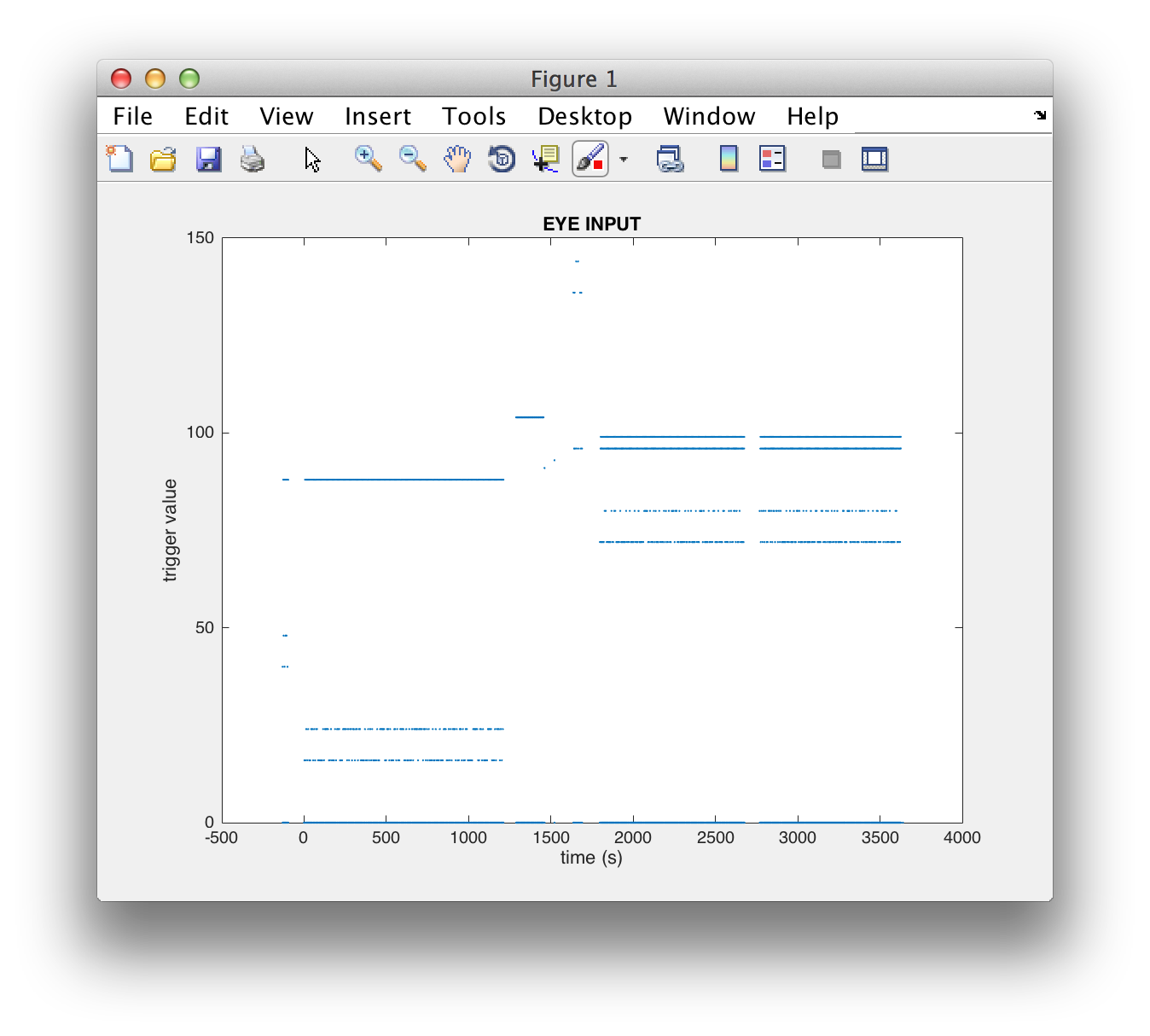You can also visualise the eye-tracker data in combination with the INPUT triggers using ft_databrowser

``````cfg = [];
cfg.viewmode       = 'vertical';
cfg.preproc.demean = 'yes';
cfg.event          = event_eye;
ft_databrowser(cfg, data_eye);
``````

or by reading the data straight from dis

``````cfg = [];
cfg.dataset = filename_eye;
ft_databrowser(cfg);
``````

The following shows the data and the events in the first 20 seconds.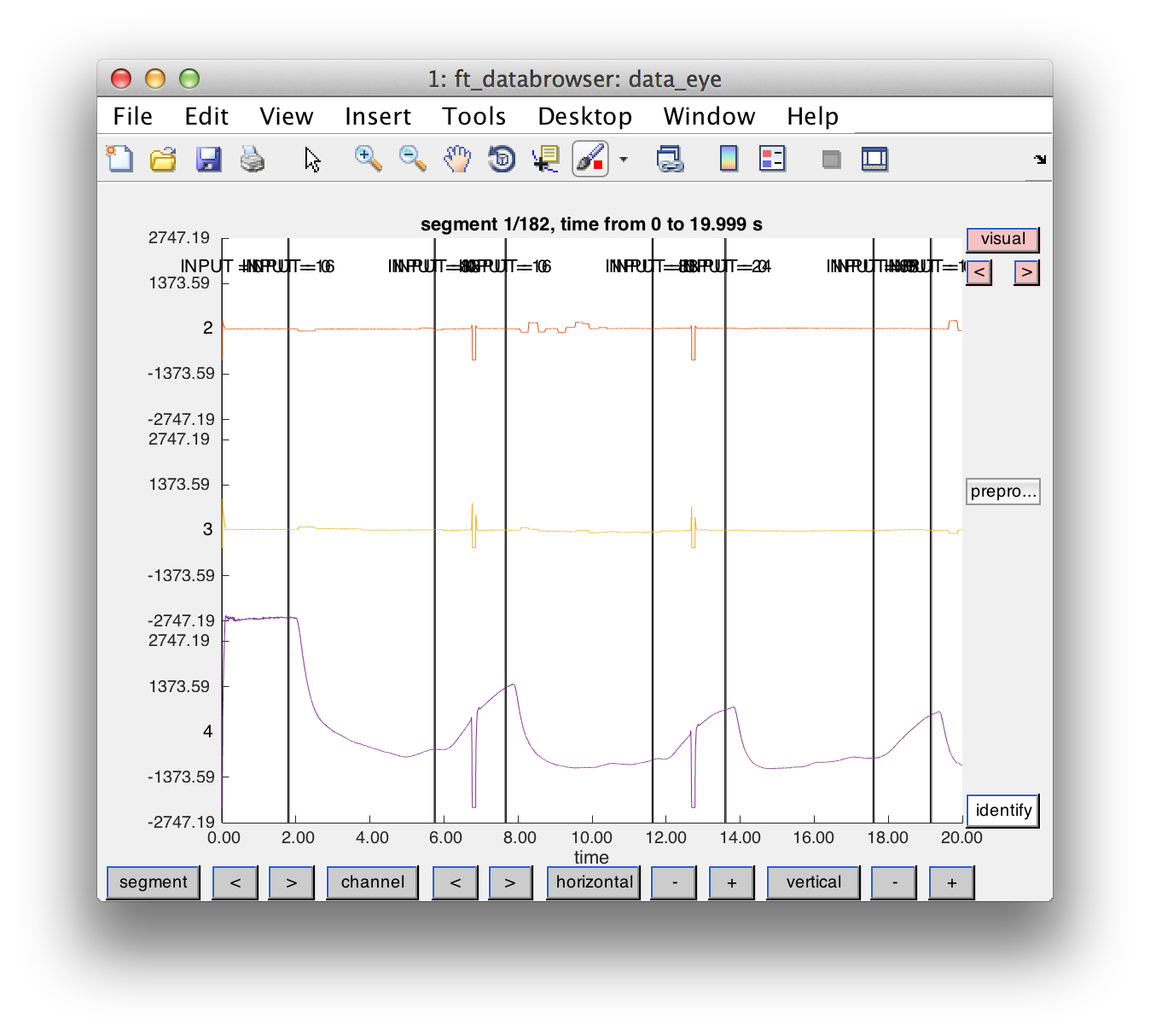### Alternative ways of importing the data

You would also try an alternative to the EDF2ASC.EXE conversion that I found here. There is also direct method, using a mex file that reads *.EDF files directly into MATLAB structures (samples and events), compiled versions for macOS and Win64 are available here. You will have to combine this with your own code to convert the data to FieldTrip format.

## Simultaneous EEG and eye-tracker recordings

If you record EEG together with eye-tracker data, you will have two separate recordings in separate files. The recordings will have been started at slightly different moments and may be a few seconds to a few minutes shifted relative to each other, depending on your experimental procedure. Furthermore, the sampling rate will be different. Hence you cannot directly append or concatenate the two datasets. The trick to link these recordings to each other is that you have to ensure that your stimulus triggers are send (and acquired by) both the EEG system and by the Eyelink system.

In the following example we are going to emulate such a situation, by reading the EEG channels from a MEG dataset, but ignoring the MEG specifics.

``````% read the EEG data, this could also come from an EEG dataset
cfg = [];
cfg.dataset = filename_eeg;
cfg.channel = {'EEG*'};
cfg.continuous = 'yes';
data_eeg = ft_preprocessing(cfg);
``````

Subsequently you can read the events from the EEG dataset.

``````event_eeg = ft_read_event(filename_eeg);

disp(unique({event_eeg.type}));

'UPPT001'    'UPPT002'    'frontpanel trigger'    'trial'
``````

In this dataset (since it is a MEG dataset) there are four types of events. The ‘trial’ and ‘frontpanel trigger’ events are not interesting; the UPPT001 events represent stimuli and the UPPT002 events represent the responses on the button box.

``````% select the UPPT001 and UPPT002 events
event_eeg_stim = event_eeg(strcmp('UPPT001', {event_eeg.type}));
event_eeg_resp = event_eeg(strcmp('UPPT002', {event_eeg.type}));

figure
plot([event_eeg_stim.sample]./data_eeg.hdr.Fs, [event_eeg_stim.value], '.')
title('MEG STIM')
xlabel('time (s)');
ylabel('trigger value');

figure
plot([event_eeg_resp.sample]./data_eeg.hdr.Fs, [event_eeg_resp.value], 'ro')
title('MEG RESP')
xlabel('time (s)');
ylabel('trigger value');
``````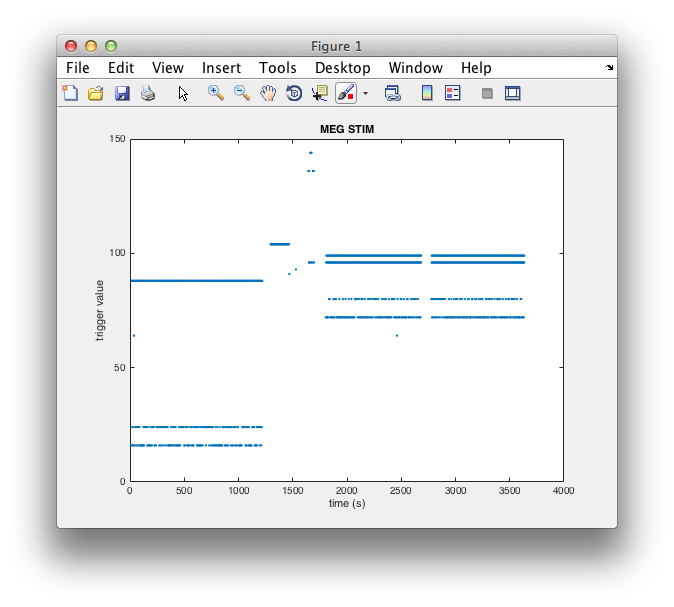Important to notice here is that the stimulus events in the EEG dataset largely correspond to the events in the eye-tracker dataset. The response events however are not represented in the eye-tracker dataset. Furthermore, the exact number of stimuli in the Eyelink data is approximately double the number of stimulus events in the EEG data.

``````>> event_eeg_stim

event_eeg_stim =

1400x1 struct array with field
type
sample
value
offset
duration

>> event_eye

event_eye =

1x2822 struct array with field
type
sample
timestamp
value
duration
offset
``````

There are 1400 triggers in one, and 2822 triggers in the other. This is mostly explained by each trigger onset and offset being represented in the Eyelink events, but only the onsets being represented in the CTF events.

There are 2 triggers in the CTF file, that are not specified anywhere and should not be there (with a value of 64). These are not present in other subjects’ data sets from the same experiment. I have no clue where they would come from but suspect it to be a hardware glitch of the Bitsi box (which links the serial port of the presentation computer with the input of the CTF and Eyelink acquisition systems). The Eyelink and CTF system, might have different detection thresholds and different minimum durations of the TTL pulse, therefore it might show up in one dataaset and not the other.

There are 9 triggers (without doubling) in the EDF explained by the fact that the recording of the EDF file, but not the MEG file, includes practice trials (because I usually start the recording of the EDF during practice to check whether the eye tracking looks okay).

There are then still 2 surplus Triggers with a value of 0 in the EDF file, which appear at the start and the end of the EDF. These are software generated and hence not in the CTF dataset.

Again using the ft_databrowser you can check the data relative to the events.

``````cfg = [];
cfg.dataset = filename_eeg;
cfg.continuous = 'yes';
cfg.channel = 'EEG';
cfg.viewmode = 'vertical';
cfg.preproc.demean = 'yes';
ft_databrowser(cfg);
``````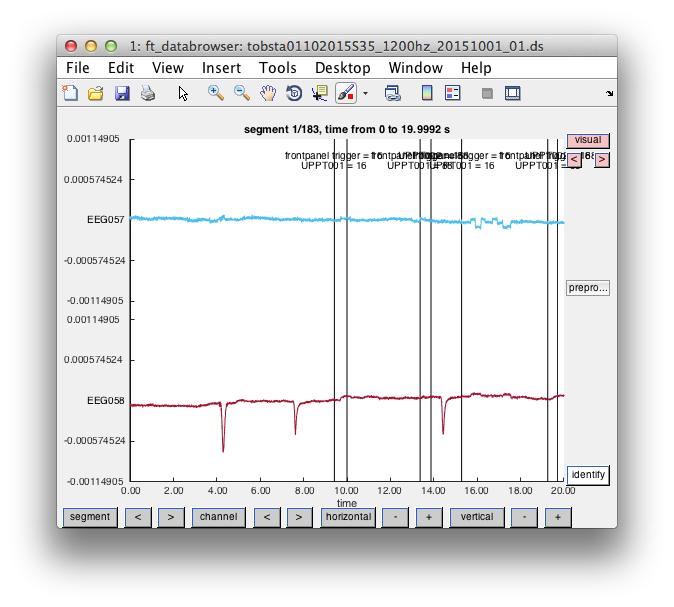To do a combined analysis of the eye-tracker and the EEG data, you would use the stimulus triggers that are present in both. Using a trial function and ft_definetrial you would do ft_preprocessing on both eye-tracker and EEG data, cutting out exactly the same segment of data around each event of interest.

``````cfg = [];
cfg.trialfun = 'your_custom_trialfun';
cfg.dataset = filename_eye;
cfg = ft_definetrial(cfg);

data_eye = ft_preprocessing(cfg);

cfg = [];
cfg.trialfun = 'your_custom_trialfun'; % this could be the same one
cfg.dataset = filename_eeg;
cfg = ft_definetrial(cfg);

data_eeg = ft_preprocessing(cfg);
``````

Subsequently you resample or interpolate the data of one recording on the time axis of the other, and you append the tw

``````cfg = [];
cfg.time = data_eeg.time;
data_eye_resampled = ft_resampledata(cfg, data_eye);

cfg = [];
data_combined = ft_appenddata(cfg, data_eeg, data_eye_resampled);
``````

## Simultaneous MEG and eye-tracker recordings

The Eyelink system has an optional Digital-to-Analog converter (DAC) card, which makes the eye position and pupil diameter available as analog signals. These analog signals can subsequently be sampled and recorded with another data acquisition system. At the DCCN we have the Eyelink DAC output connected to the CTF275 general-purpose ADC channels. The synchronization between the eye-tracker and the MEG data is trivial in this case, given that both data types are sampled by the MEG electronics. In this case you do not need the ASC or EDF file, although we nevertheless recommend that you copy them from the Eyelink computer and archive them along with your MEG data.

``````cfg = [];
cfg.dataset = filename_meg;
data_meg = ft_preprocessing(cfg);
``````

Again, using the procedure as described for the EEG, you can combine the recordings of the Eyelink system and the MEG.

The DAC conversion in the Eyelink system takes some time, and therefore the UADC channels in the MEG recording have a small (but fixed) delay relative to the actual eye movements.

Since both MEG and Eyelink get the same triggers, you can use FieldTrip ft_definetrial on both to read the same segments.

``````cfg = [];
cfg.dataset = filename_meg;
cfg.trialdef.eventtype      = 'UPPT001';
cfg.trialdef.eventvalue     = 104;
cfg.trialdef.prestim        = .5;
cfg.trialdef.poststim       = 1;
cfg.continuous  = 'yes';
cfg = ft_definetrial(cfg);
data_meg = ft_preprocessing(cfg);

cfg = [];
cfg.dataset = filename_eye;
cfg.trialdef.eventtype      = 'INPUT';
cfg.trialdef.eventvalue     = 104;
cfg.trialdef.prestim        = .5;
cfg.trialdef.poststim       = 1;
cfg = ft_definetrial(cfg);
data_eye = ft_preprocessing(cfg);
``````

and plot them side by side

``````uadc005 = find(strcmp(data_meg.label, 'UADC005'));

figure
subplot(2,1,1)
plot(data_eye.time{2}, data_eye.trial{2}(2,:))
grid on

subplot(2,1,2)
grid on
``````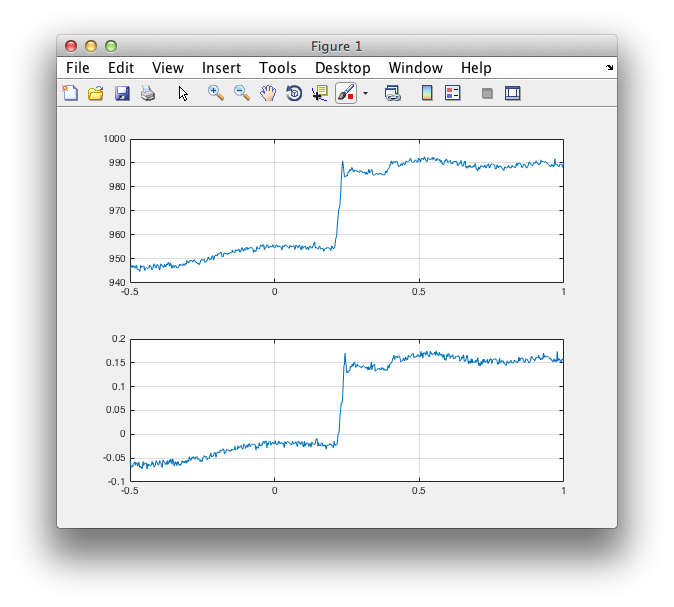If you look carefully, you can see the delay in the MEG ADC channels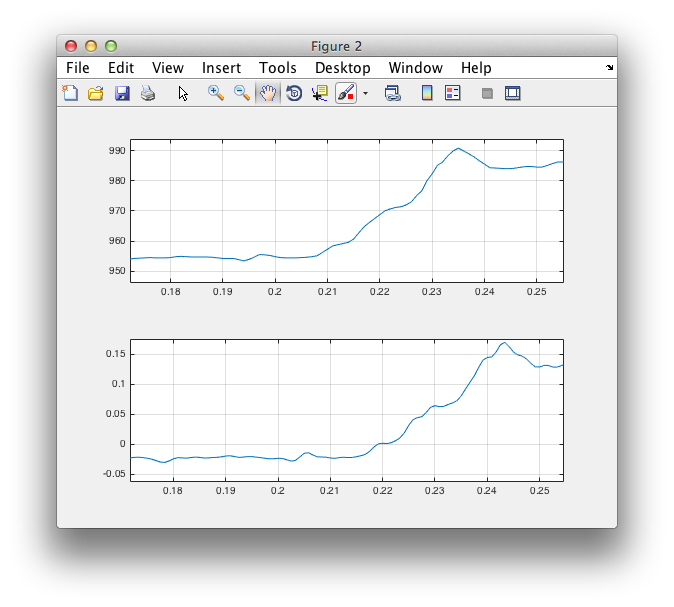Subsequently you can resample the 1000Hz Eyelink data to the 1200Hz MEG data and append them in the same data structure for more convenient joint processing.

``````cfg = [];
cfg.time = data_meg.time;
data_eye_resampled = ft_resampledata(cfg, data_eye)

cfg = [];
data_combined = ft_appenddata(cfg, data_meg, data_eye_resampled);
``````

## What are the units of the eye-tracker data?

The units of the eye-tracker data depend on the specifications in the ‘Set Options’ screen of the Eyelink acquisition software. Here, the units of GAZE output for horizontal (x-coordinate) and vertical (y-coordinate) data are described.

Eye position data can in principle be described as

• viewing direction, expressed in degrees visual angle

• position on screen, expressed in pixels You can convert between these two representations using some trigonometry. For small angles and centre positions, they are approximately linearly related, but not for more eccentric positions. You should also keep the offset in mind, i.e. the angle or position which is defined as (0,0). Visual angle is most conveniently expressed relative to the center of the screen (i.e. the fixation point), whereas position on screen is most conveniently expressed relative to the upper left corner as pixel (0,0).

### MEG data - UADC channels

In the Eyelink ‘Set Options’ screen, set ‘Analog Output’ to ‘GAZE’.

The UADC channel values are expressed in Volt. The GAZE positions as recorded in the EDF file are converted into voltages according to these formula

``````R     = (voltage - minvoltage)/(maxvoltage - minvoltage)
S     = R*(maxrange - minrange) + minrange
Xgaze = S*(screenright  - screenleft + 1) + screenleft
Ygaze = S*(screenbottom - screentop  + 1) + screentop
``````

The minimum/maximum voltage range and the maximum/minimum range of the data are defined in EyeLink configuration file FINAL.INI. The physical dimensions of your screen (screenright, screenleft, screenbottom, screentop) are defined in PHYSICAL.INI, or your presentation settings.

Make sure that you used calibration and validation for meaningful GAZE output!

This is an example how to convert the horizontal and vertical traces from the UADC channels in Volt to screen coordinates in pixel

``````Xgaze=[];
Ygaze=[];
for trln=1:size(data_meg.trial,2)

R_h = (voltageH-minvoltage)./(maxvoltage-minvoltage); %voltage range proportion
S_h = R_h.*(maxrange-minrange)+minrange; %proportion of screen width or height

R_v = (voltageV-minvoltage)./(maxvoltage-minvoltage);
S_v = R_v.*(maxrange-minrange)+minrange;

S_h = ((voltageH-minvoltage)./(maxvoltage-minvoltage)).*(maxrange-minrange)+minrange;
S_v = ((voltageV-minvoltage)./(maxvoltage-minvoltage)).*(maxrange-minrange)+minrange;

Xgaze(trln,:) = S_h.*(screenright-screenleft+1)+screenleft;
Ygaze(trln,:) = S_v.*(screenbottom-screentop+1)+screentop;

end
``````

### EDF data - position

In the ‘Set Options’ screen, set ‘File Sample Contents’ to ‘GAZE position’.

Gaze position data reports the actual (x, y) coordinates of the subject’s gaze on the display, compensating for distance from the display. The units are in actual Data Files display coordinates (usually pixels) which can be set in the EyeLink configuration file PHYSICAL.INI. The default EyeLink coordinates are mapped to a 1024x768 display, with (0,0) at the top left and (1023,767) at the bottom right. Note that the settings of your presentation screen and the presentation software may overwrite the settings in PHYSICAL.INI.

### EDF data - pupil

Pupil size data can be recorded as ‘area’ or ‘diameter’ in pixels. The area is recorded in scaled image pixels. Diameter is calculated from pupil area fit using a circle model. Note that when pupil diameter is your variable of interest, you should correct for viewing direction. When the eye rotates away from the camera during viewing, the pupil seems smaller. This effect can be corrected for with a geometrical model described by Hayes and Petrov (2016), but this requires that you know the geometry of the eye tracking camera relative to the eye and the computer screen. Alternatively, you could use ft_regressconfound to try and remove the influence of viewing direction on pupil diameter.

### Mapping to Presentation Stimuli

If you want to inspect the accuracy of the recorded Gaze positions compared to what you presented on the screen, you need to convert the Presentation coordinates to screen coordinates. The convention in Presentation is that {x = 0; y = 0;} refers to the center of the screen. In case of even screen width and height, Presentation assumes an extra Pixel, i.e., in that case Presentation’s central pixel is one pixel to the right and one pixel below the true screen center.

An example for mapping between Gaze postions and presented stimuli (trials 1 to 5) is shown below (+ indicates presented targets, Gaze position depicted in blue).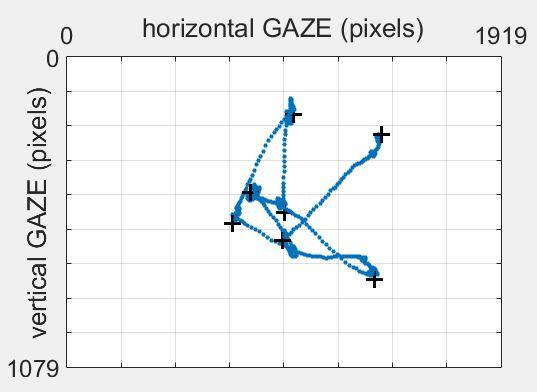In this example, a 1920 x 1080 screen was used.